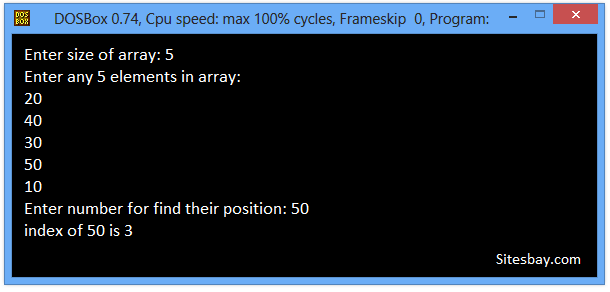# Search Array Elements Program in C++

## Search an Array Elements Program in C++

For search array element you need to compare all array element with that particular elements. In below C++ program we have to search an element in a given array using linear search algorithm. If given element is present in array then we will print it's index otherwise print a message saying element not found in array.

To understand this code you need basic knowledge about Array in C++ and For Loop in C++.

### Linear Search in C++

In linear search algorithm, we compare targeted element with each element of the array. If the element is found then its position is displayed. The worst case time complexity for linear search is O(n).

## Example

```Input Array : [6, 12, 9, 8, 31, 10, 25]
Element to search : 31
Output :
Element found at index 4
```

## Search Array Elements Program in C

```#include<iostream.h>
#include<conio.h>

void main()
{
int i,a,num,size;
clrscr();
cout<<"Enter size of array: ";
cin>>size;
cout<<"Enter any"<<size <<"element in array: \n";
for(i=0; i<size; i++)
{
cin>>a[i];
}
cout<<"Enter number for find their position: ";
cin>>num;
for(i=0; i<size; i++)
{
if(a[i]==num)
{
cout<<"\n index of "<<num <<" is " <<i;
}
}
getch();
}
```

## Output

```Enter size of array: 5
Enter any 5 elements in array:
20
40
30
50
10
Enter number for find their position: 50
index of 50 is 3
```

Note: Here we print number inxed value. That's why actual position of number is less than 1.## Pure VPN Privide Lowest Price VPN Just @ \$1.65. Per Month with Non Detected IP Lowest Price Non Detected IP VPN